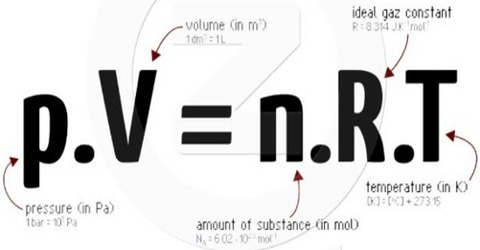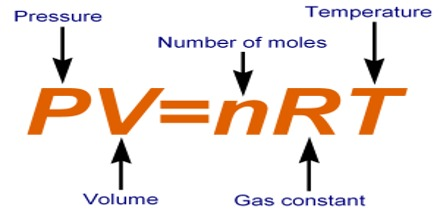Chemistry

# Ideal Gas EquationThe Ideal Gas Equation

Boyle’s, Charle’s and Avogadro’s laws may be combined to give a general relation between the V, P, T and n of a gas. Such a general relation is known as an equation of state. The equation of state shows how P, V, T and n are interrelated.

The equation of state for an ideal gas may be deduced as follows:

Let P = pressure, V= volume, T = absolute temperature and n = number of moles of any gas.

We have seen that;

• V ∞ 1/P [at constant T and n (Boyle’s Law)]
• V ∞ T [at constant P and n (Charle’s Law)]
• V ∞ n [at constant P and T (Avogadro’s Law)]

From the laws of variation, it follows that,

V ∞ (1/P).T.n

Or, V ∞ R.(nT/P); where R is the Constant.

Rearranging this equation, we get,

PV = nRTThis is the equation of state for an ideal gas. It applies to all gases. The word ‘ideal’ is used because there is no actual gas whose behavior follows this equation strictly under all conditions of temperature and pressure. The relationship is, however, useful in most calculations involving gases where high precision is not necessary.